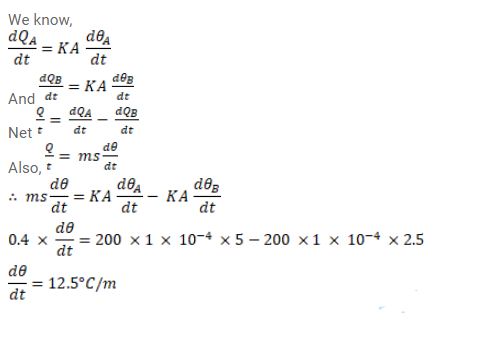# A metal rod of cross - sectional areaQuestion:

A metal rod of cross - sectional area $10 \mathrm{~cm}^{2}$ is being heated at one end. At one time, the temperature gradient is $5.0^{\circ} \mathrm{C} / \mathrm{cm}$ at cross - section $\mathrm{A}$ and is $2.5^{\circ} \mathrm{C} / \mathrm{cm}$ at cross-section $\mathrm{B}$. Calculate the rate at which the temperature is increasing in the part $\mathrm{AB}=0.40 \mathrm{~J} /{ }^{\circ} \mathrm{C}$, thermal conductivity of the material of the rod = $200 \mathrm{~W} / \mathrm{m}^{\circ}{ }^{\circ} \mathrm{C}$. Neglect any loss of heat to the atmosphere.

Solution: